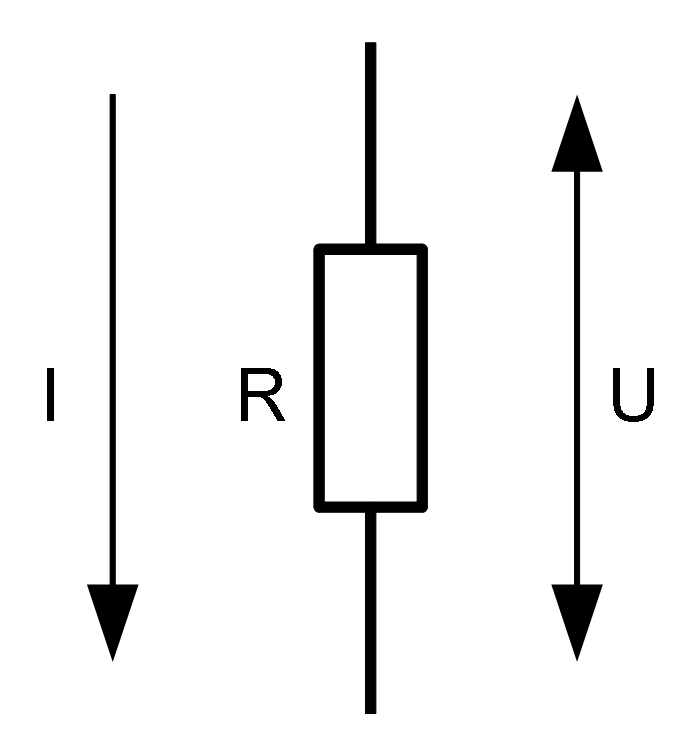# Ohm's Law

Ohm's law states that the current through a conductor between two points is directly proportional to the potential difference or voltage across the two points, and inversely proportional to the resistance between them, provided that the temperature remains constant.

The equation is as follows:Resistance of the conductor, applied voltage and current through the resistance

I = U / R

where:

• I is current
• U is voltage
• R is resistance

Several other equations that are used often in electronics are derived from Ohm's law.